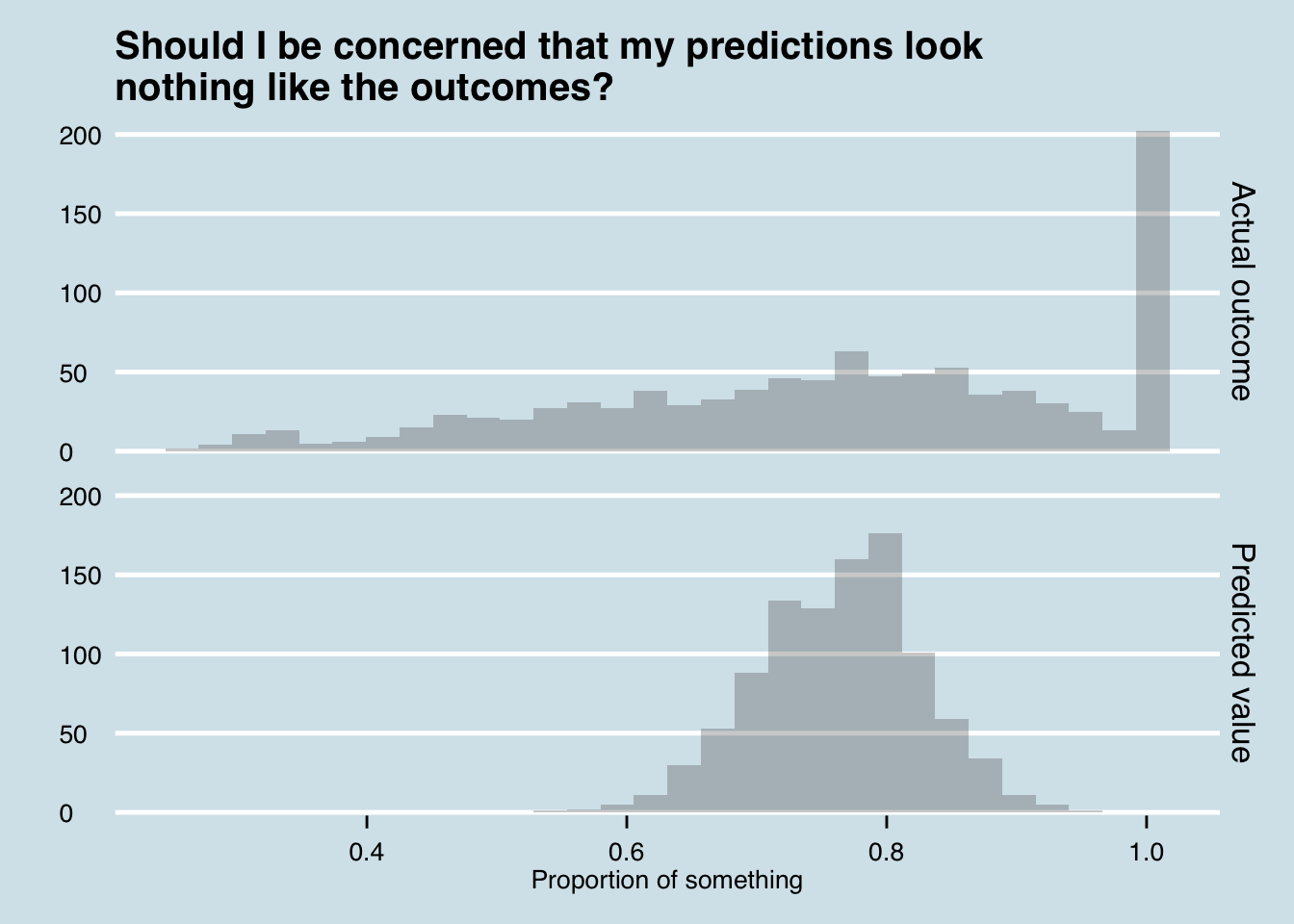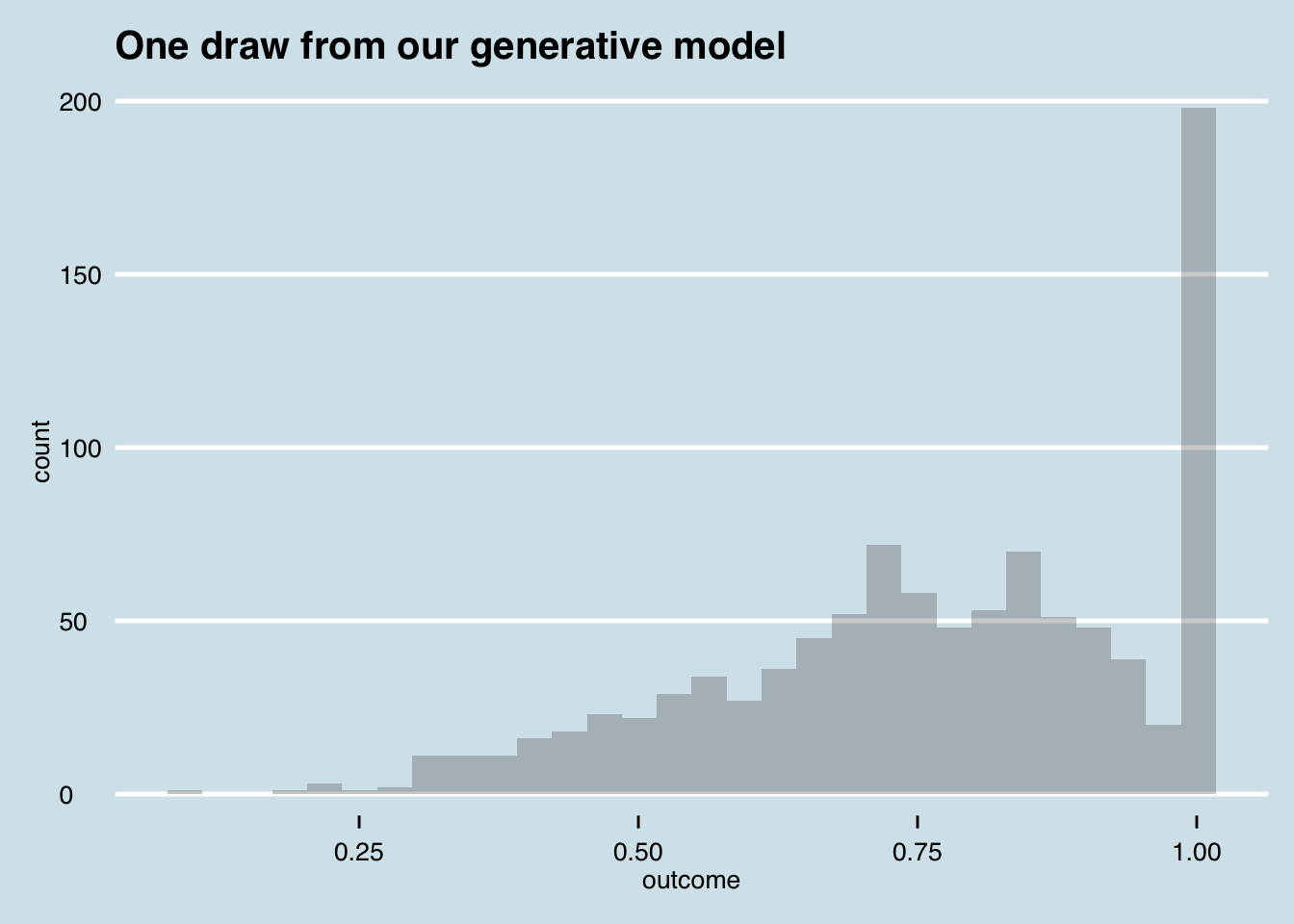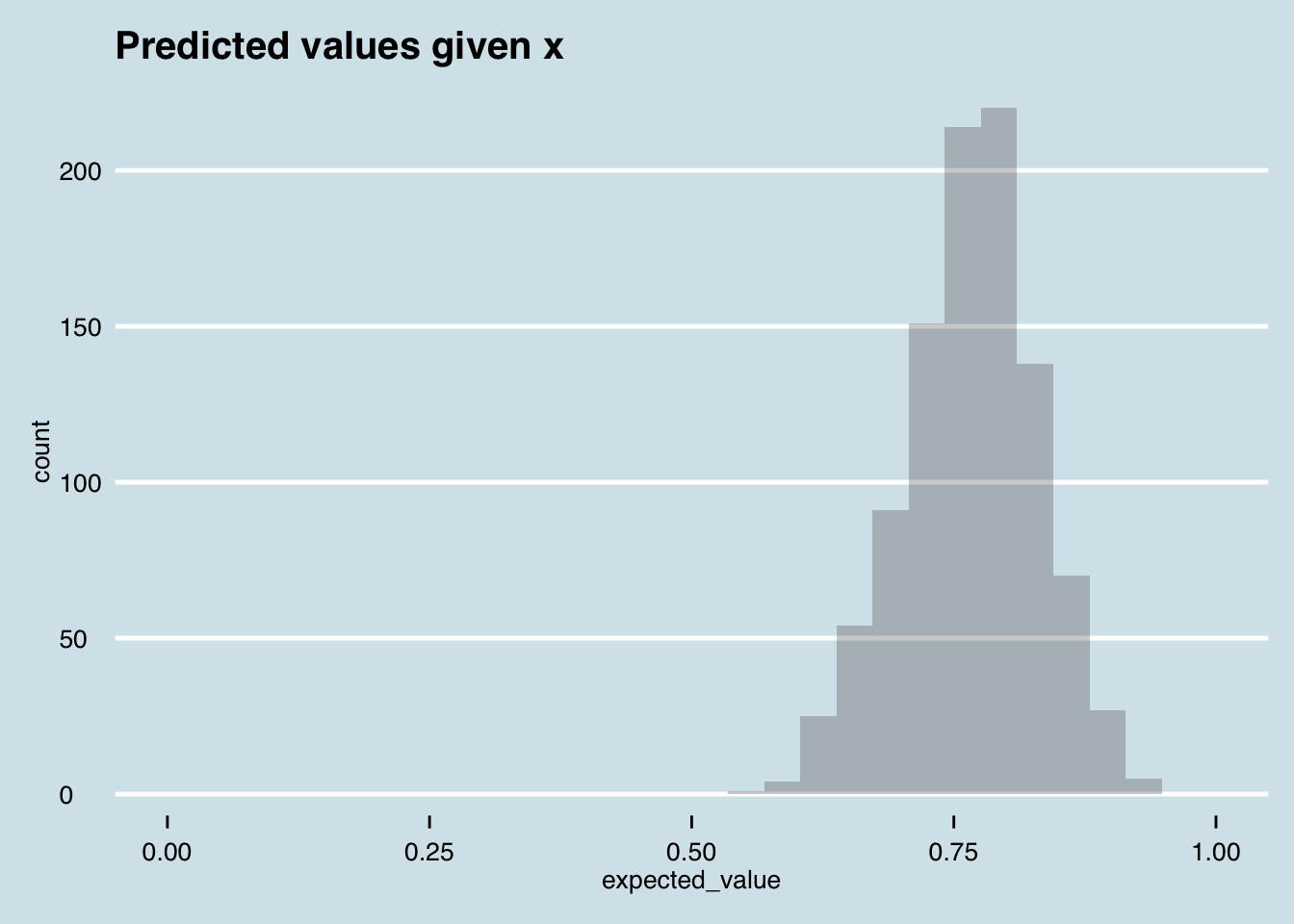## Monday, August 29, 2016

### Why you should be posterior predictive checking

This week at work I had an excellent problem come across my desk. A client, for whom we had done some modeling work, sent across a plot that looked a little like the one below (this is all fake data).
The client wanted to know if they should be concerned that the distribution of actual outcomes (the top panel) look nothing like the predictions (bottom panel).If you’ve never played with models before and you saw this chart, your natural response might be to think that the model was terrible. And a terrible model might produce predictions that look nothing like the actual outcomes. Yet in this case, you would be mistaken in writing off the model due to the difference in plots. The predictions in the chart above actually come from the exact same model that I used to generate the “Actual outcome” data—it is impossible to get a better set of predictions than those summarized in the bottom panel. So what’s going on? Let’s step through it.
Often when modeling proportions, we get weird-looking distributions like the top panel in the chart above. Often there are spikes at 0 and 1, and some distribution of continuous outcomes between. In this particular example, we have a spike at 1 and a set of proportons roughly between 0.3 and 0.9, skewed towards higher values in this range.
A popular way of modeling these distributions is to break up the problem using a generative model. A generative model asks “what is a plausible mathematical structure that could give rise to the data that we observe.” One way of breaking up the problem would be to consider there actually being two processes.
1. The first process determines whether an observation is 1 or less than 1.
2. If the proportion is not 1, the second process would assign relative probabilities to outcomes in the range (0,1).
Let’s get specific. If ${\beta }_{2}$, and ${\beta }_{3}$ are coefficient vectors, , and  are intercept terms and X is a design matrix containing our predictive features, then we might say that the data generating process for an outcome ${Y}_{i}$ could be
Step 1:


where  is the indicator function, and Step 2, in the case that :


For those who’ve not used them before, the  density is a highly flexible distribution that takes two positive parameters and assigns weight to observations between 0 and 1, with no probability of outcomes outside that interval. The wikipedia entry is helptul.
A nice thing about using a model like this to model proportions is that it assigns some weight to 1 for all values of , and weight between 0 and 1 otherwise. If you had a spike at 0 also, you could simply replace the first model with a multinomial logit model, and it’d have the same benefits.

## Simulating a draw from the model

When we build generative models, we consider the world as observed to be just a single draw from a universe of different potential draws. Our generative model is just a probability density over those potential draws. So we can generate one draw from the model as so (in R).
# Load the relevant libraries
library(reshape2); library(arm); library(ggplot2); library(dplyr)

# Set some known parameter values

# We'll just assume a single covariate x

# parameters for the logit
beta11 <- -1.5
beta12 <- 0.5

# parameters for the beta
beta21 <- 5
beta22 <- 0.5
beta31 <- 2
beta32 <- -0.3

# Number of observations
N <- 1000

# Generate our predictor x
x <- rnorm(N)

# Generate some draws from the beta distribution for the continuous outcome
continuous <- rbeta(N, beta21 + beta22*x, beta31 + beta32*x)

# Generate the probabilities for the binary outcome
prob_binary <- invlogit(beta11 + beta12*x)

# Generate the draws for the binary outcome
binary <- rbinom(N, size = 1, prob = prob_binary)

# The outcome as defined
outcome <- ifelse(binary==1, binary, continuous)
Now we can plot the fake outcome data that we’ve created.
data_frame(outcome) %>%
ggplot(aes(x = outcome)) +
geom_histogram(alpha = 0.3) +
ggthemes::theme_economist() +
ggtitle("One draw from our generative model")So now let’s generate predictions for each value of  for the value of . How might we do this? Two methods could work.
• The first method works when we have analytically tractable models. We can simply calculate the expected value of  for each value of  using basic algebra.
• The second method is what we do when we have larger, more complex models for which analytical expected values might be hard to derive. In this approach, we’d simulate the model hundreds or thousands of times for each value of , and take the average of the simulated outcomes.
In this toy example, we will do the first. It happens that the epected value from a Beta distribution with parameters  is . The expected value from a Bernoulli logit model is simply the probability, here . So for a given value of , our prediction is just the weighted average of the two models:

Now if we plot this density, we get something that looks nothing like our data density. Why? The expected value averages across two outcomes in proportion to their probability, so only those observations with an extremely high probability of having a proportion equal to 1 will have an expected value anywhere near 1.
expected_value <- (1 - prob_binary)*((beta21 + beta22*x)/(beta21 + beta22*x+ beta31 + beta32*x)) + prob_binary

data_frame(expected_value) %>%
ggplot(aes(x = expected_value)) +
geom_histogram(alpha = 0.3) +
ggthemes::theme_economist() +
ggtitle("Predicted values given x") +
xlim(0, 1)## So if I can’t validate my model by comparing actual and predicted distributions, what can I do?

In three words, posterior predictive checking. This method involves simulating outcomes from your model, incorporating any uncertainty you have about the parameters of the model after estimating it. The steps
1. Estimate your model. If you are a Bayesian (you should be), and performed MCMC, you will have hundreds or thousands of draws from the joint density of , in proportion to their posterior probability. If you are a frequentist, you should have performed some magic to get many draws from the parameter space (I’m not sure this is actually possible). Common techniques would be bootstrapping or making distributional assumptions about the parameters.
2. For each parameter draw from your model, for each value of , draw an outcome from the model. These draws make up your posterior predictions.

## A worked exercise

Let’s use the realized  and  to estimate this model in Stan and generate posterior predictive draws.
# A Stan program to estimate the model described above

beta_logit_model <- "
data {
int N; // number of observations
int P; // number of explanatory variables
int N2; // number of observations to predict
vector[N] Y; // the proportion paid of expected at some period ahead
matrix[N, P] X; // The explanatory variables (make sure no variables known in the future!)
matrix[N2, P] X_new; // explanatory variables for out-of-sample
}
transformed data {
// we an integer Y and a real Y (Y_adj is only here for flexibility of extension)
int Y_int[N];

for(i in 1:N) {
if(Y[i] == 1.0) {
Y_int[i] = 1;
} else {
Y_int[i] = 0;
}
}
}
parameters {
matrix[3, P] beta;
vector mu;
}
model {
// priors
to_vector(beta) ~ normal(0, .2);
mu ~ normal(0, 1);

// likelihood
Y_int ~ bernoulli_logit(mu + X*beta');

for(i in 1:N) {
if(Y_int[i]==0) {
// We constrain alpha and beta to be positive by taking exp()

Y_adj[i] ~ beta(exp(mu + X[i]*beta'),exp(mu + X[i]*beta'));
}
}
}
generated quantities {
vector[N2] Y_predict;
int tmp;
for(i in 1:N2) {
tmp = bernoulli_rng(inv_logit(mu + X_new[i]*beta'));
if(tmp ==1) {
Y_predict[i] = 1.0;
} else {
Y_predict[i] = beta_rng(exp(mu + X_new[i]*beta'), exp(mu + X_new[i]*beta'));
}
if(is_nan(Y_predict[i])) {
Y_predict[i] = 1.1;
}
}
}

"
Now we estimate the model
library(rstan)
options(mc.cores = parallel::detectCores())

beta_logit_estimation <- stan(model_code = beta_logit_model,
data = list(N = N,
P = 1,
N2 = N,
Y = outcome,
X = matrix(x, N, 1),
X_new = matrix(x, N, 1)),
iter = 600)
And finally plot the posterior replicatons
predictions <- as.data.frame(beta_logit_estimation) %>%
select(contains("Y_predict")) %>%
melt()

predictions %>%
ggplot() +
geom_line(aes(x = value, group = variable), colour = "orange", stat = "density", alpha = 0.1, adjust = .8) +
geom_density(data = data_frame(outcome), aes(x = outcome), colour = "black", adjust = 0.8) +
ggthemes::theme_economist() +
ggtitle("Actual outcomes and posterior predictive replications") +
annotate("text", x = 0.2, y = 5, label = "Density of actual outcomes", hjust = 0) +
annotate("text", x = 0.2, y = 3.5, label = "Posterior replications", colour = "orange", hjust = 0)